# 3 Digit Subtraction with Regrouping

3 Digit Subtraction with Regrouping – Question 1

Subtract the following: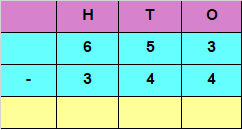Explanation

If we want to Subtract the digits at ones place, we have

3 – 4

But 4 is greater than 3

We would need to borrow 1 from 5 in tens place

3 in ones place would now become 13 and 5 in tens place would be reduced to 4

So, 13 – 4 = 9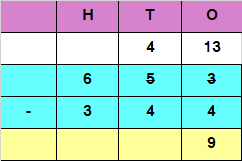Subtracting the digits at tens place

4 – 4 = 0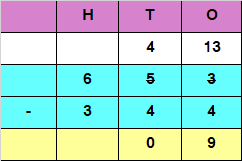Subtracting the digits at hundreds place

6 – 3 = 3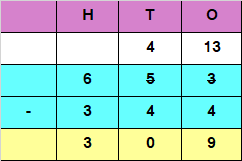Hence, the difference is 309

3 Digit Subtraction with Regrouping – Question 2

Subtract the following: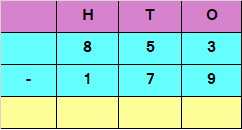Explanation

If we want to Subtract the digits at ones place, we have

3 – 9

But 9 is greater than 3

We would need to borrow 1 from 5 in tens place

3 in ones place would now become 13 and 5 in tens place would be reduced to 4

So, 13 – 9 = 4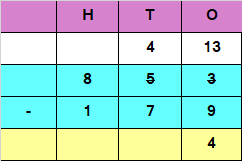If we want to Subtract the digits at tens place, we have

4 – 7

But 7 is greater than 4

We would need to borrow 1 from 8 in hundreds place

4 in tens place would now become 14 and 8 in hundreds place would be reduced to 7

So, 14 – 7 = 7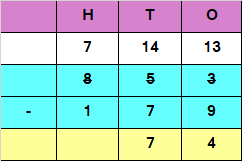Subtracting the digits at hundreds place

7 – 1 = 6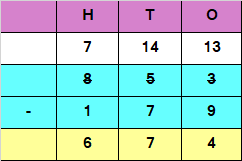Hence, the difference is 674